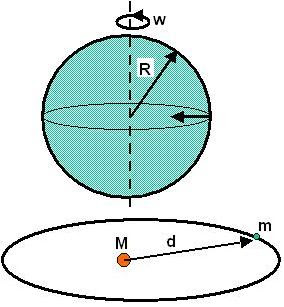## Thursday, October 2, 2008

### Irodov Problem 1.219Let the masses of Earth and Sun be m and M respectively. Let the radius of the Earth be R and the distance of the Sun from Earth be d (assuming its almost a circular orbit). Let the angular velocity of Earth be w. Then we have,
We know that,So we have,